# ggplot2 3.1.0

Mara Averick and Claus Wilke

We’re happy to announce the release of ggplot2 3.1.0 on CRAN. ggplot2 is a system for declaratively creating graphics, based on The Grammar of Graphics. You provide the data, tell ggplot2 how to map variables to aesthetics, what graphical primitives to use, and it takes care of the details.

The 3.1.0 release is a minor release that fixes a number of bugs and adds a few new features. Breaking changes have been kept to a minimum and end users of ggplot2 are unlikely to encounter any issues when switching from 3.0.0 to 3.1.0. However, there are a few items that developers of ggplot2 extensions should be aware of. For a complete list of changes and issues of relevance for extension developers, please see the release notes.

## New features

`coord_sf()` has much-improved customization of axis tick labels. Labels can now be set manually, and there are two new parameters, `label_graticule` and `label_axes`, that can be used to specify which graticules to label on which side of the plot. In particular, `label_graticule` labels the North, West, East, or South ends of the graticule lines, specified via a simple string argument. For example, `label_graticule = "NE"` will label the North and East ends, which in a standard projection with North pointing up will result in labels on top and to the right.

``````library(sf)

nc <- st_read(system.file("shape/nc.shp", package = "sf"), quiet = TRUE)

ggplot() +
geom_sf(aes(fill = AREA), data = nc) +
coord_sf(label_graticule = "NE") +
scale_y_continuous(
breaks = c(34, 34.5, 35, 35.5, 36, 36.5),
labels = parse(text = c("34 * degree * N", "NA", "35 * degree * N", "NA", "36 * degree * N", "NA"))
)
``````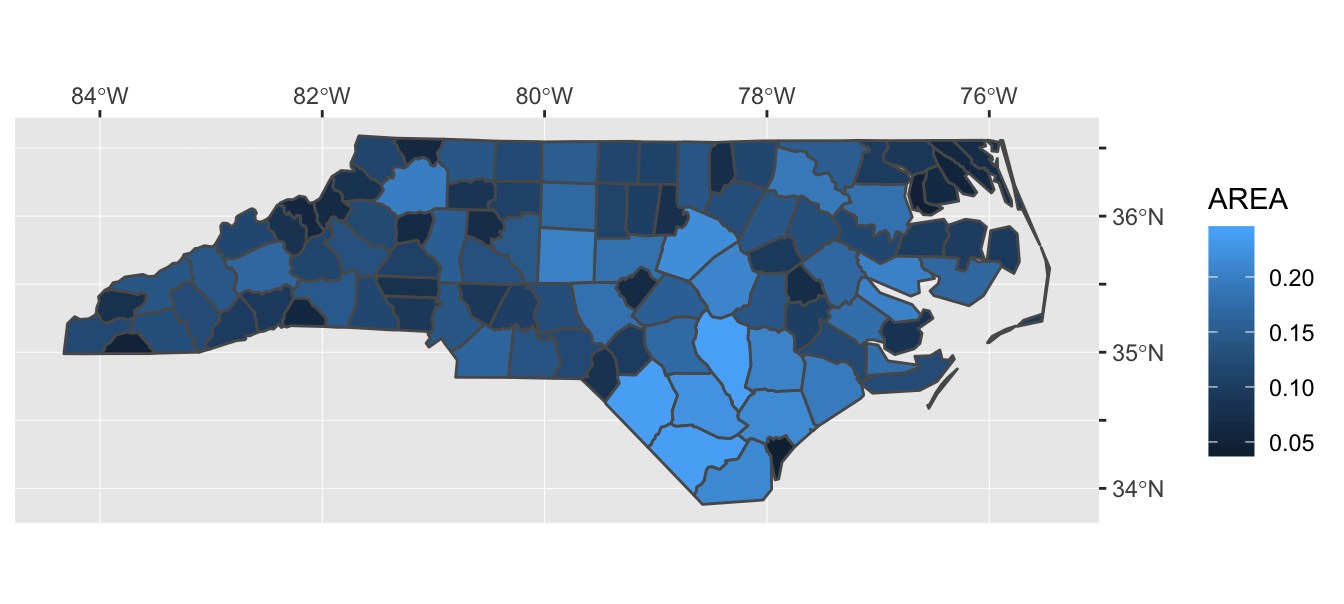Two new geoms, `geom_sf_label()` and `geom_sf_text()`, can draw labels and text on sf objects.

``````ggplot(nc[1:3, ]) +
geom_sf(aes(fill = AREA)) +
geom_sf_label(aes(label = NAME))
``````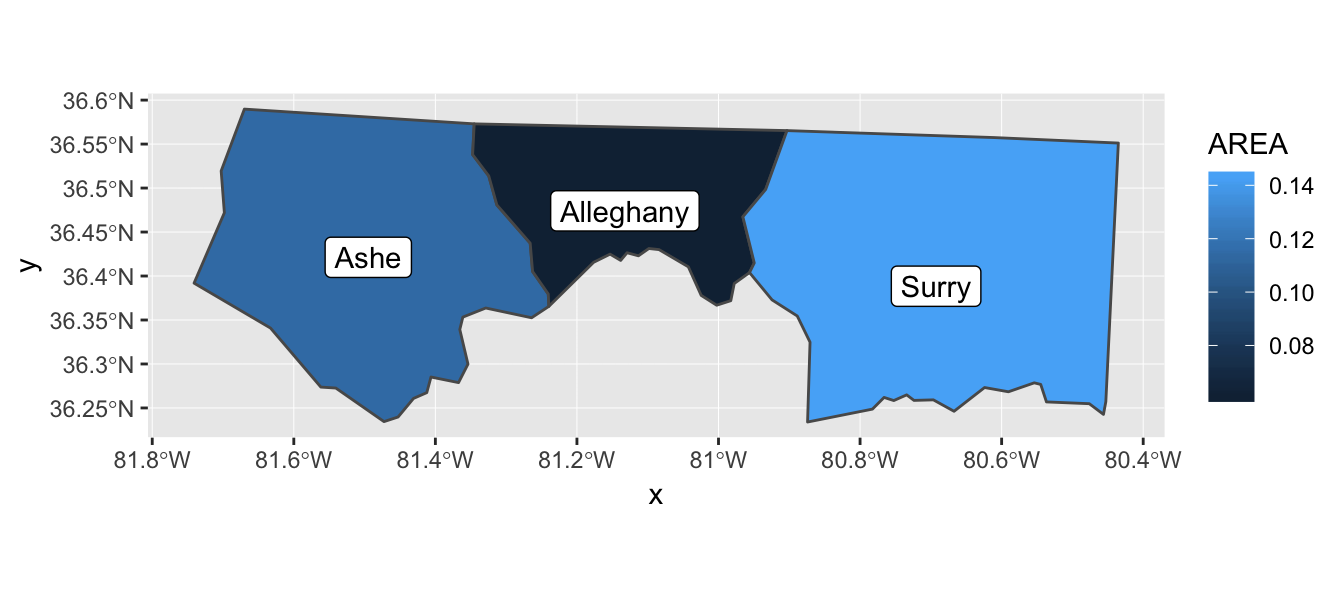Under the hood, a new `stat_sf_coordinates()` calculates the x and y coordinates from the coordinates of the sf geometries. You can customize the calculation method via the `fun.geometry` argument.

``````# default uses st_point_on_surface(), which guarantees the calculated point
# falls inside each polygon
ggplot(nc) +
geom_sf() +
stat_sf_coordinates(geom = "point", color = "red")
``````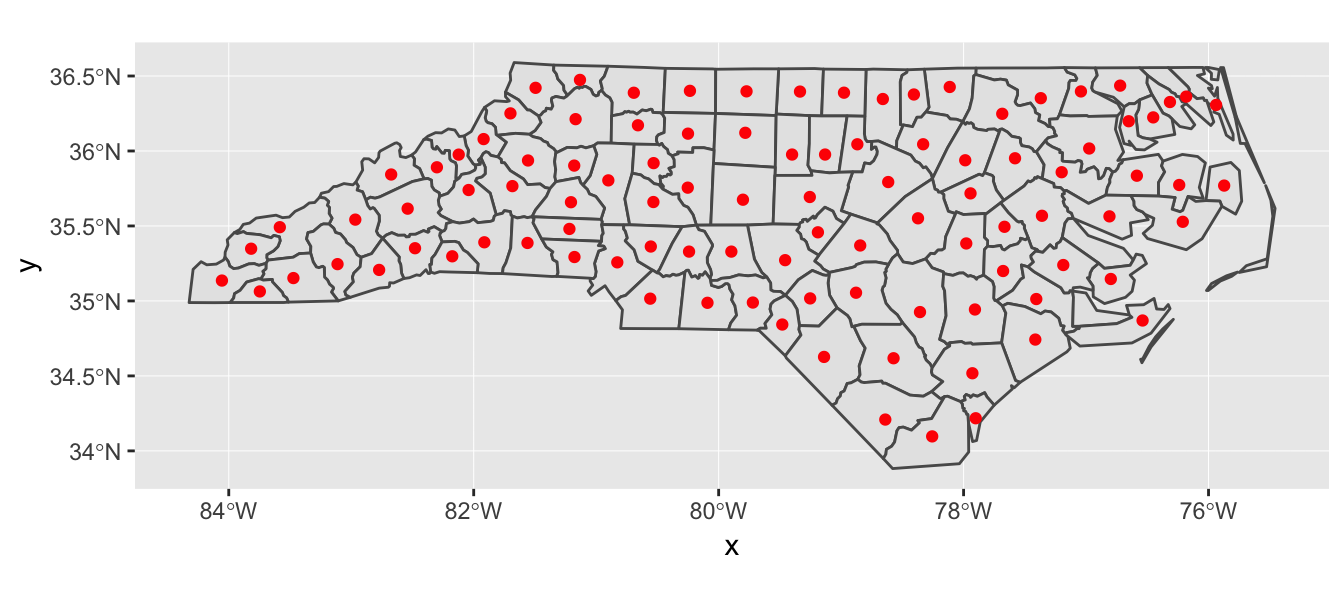``````
# can use st_centroid() instead to draw centroids
ggplot(nc) +
geom_sf() +
stat_sf_coordinates(
fun.geometry = function(x) st_centroid(st_zm(x)),
geom = "point", color = "red"
)
``````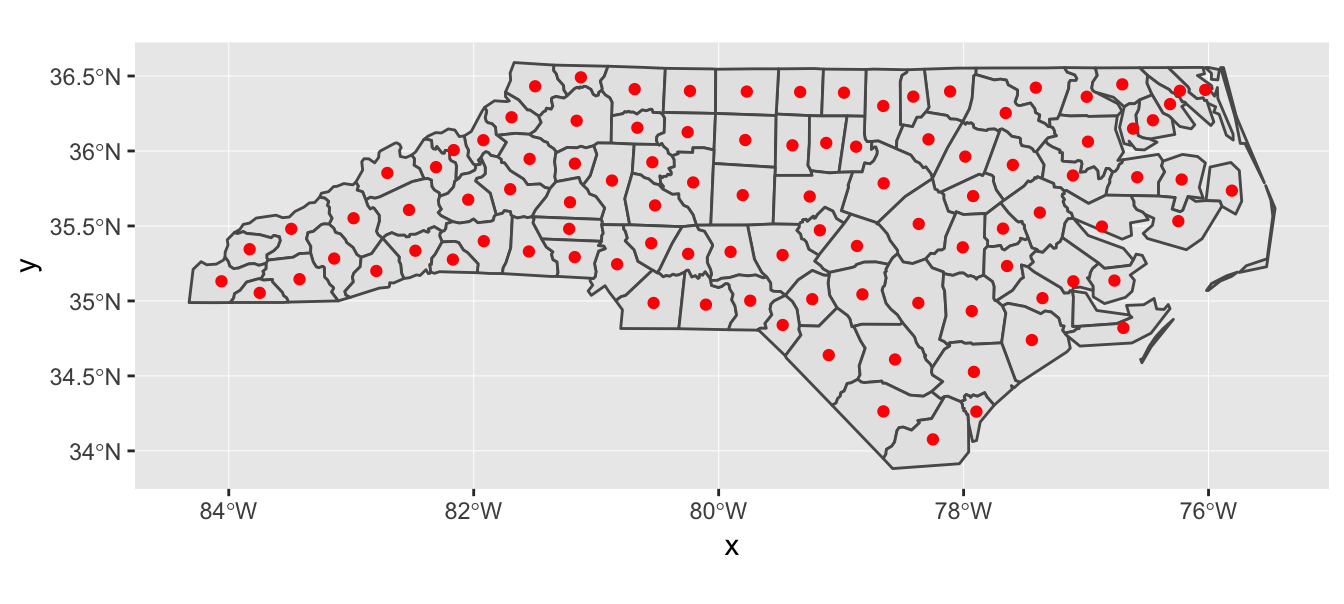## Minor fixes and improvements

Aesthetics containing the word “color” are now always standardized internally to read “colour”. This happens transparently to the end user, so that the British and American spellings of “colour” / “color” are fully equivalent throughout the entire ggplot2 code base. Therefore, the following four examples all produce the same plot.

``````p1 <- ggplot(iris, aes(x = Sepal.Length, y = Petal.Length, color = Sepal.Length, fill = Petal.Length)) +
geom_point(shape = 21, size = 3, stroke = 2) +
scale_color_viridis_c(
aesthetics = c("color", "fill"),
name = "Length", option = "B"
)

p2 <- ggplot(iris, aes(x = Sepal.Length, y = Petal.Length, colour = Sepal.Length, fill = Petal.Length)) +
geom_point(shape = 21, size = 3, stroke = 2) +
scale_colour_viridis_c(
aesthetics = c("colour", "fill"),
name = "Length", option = "B"
)

p3 <- ggplot(iris, aes(x = Sepal.Length, y = Petal.Length, colour = Sepal.Length, fill = Petal.Length)) +
geom_point(shape = 21, size = 3, stroke = 2) +
scale_colour_viridis_c(
aesthetics = c("color", "fill"),
name = "Length", option = "B"
)

p4 <- ggplot(iris, aes(x = Sepal.Length, y = Petal.Length, color = Sepal.Length, fill = Petal.Length)) +
geom_point(shape = 21, size = 3, stroke = 2) +
scale_color_viridis_c(
aesthetics = c("colour", "fill"),
name = "Length", option = "B"
)

cowplot::plot_grid(p1, p2, p3, p4)
``````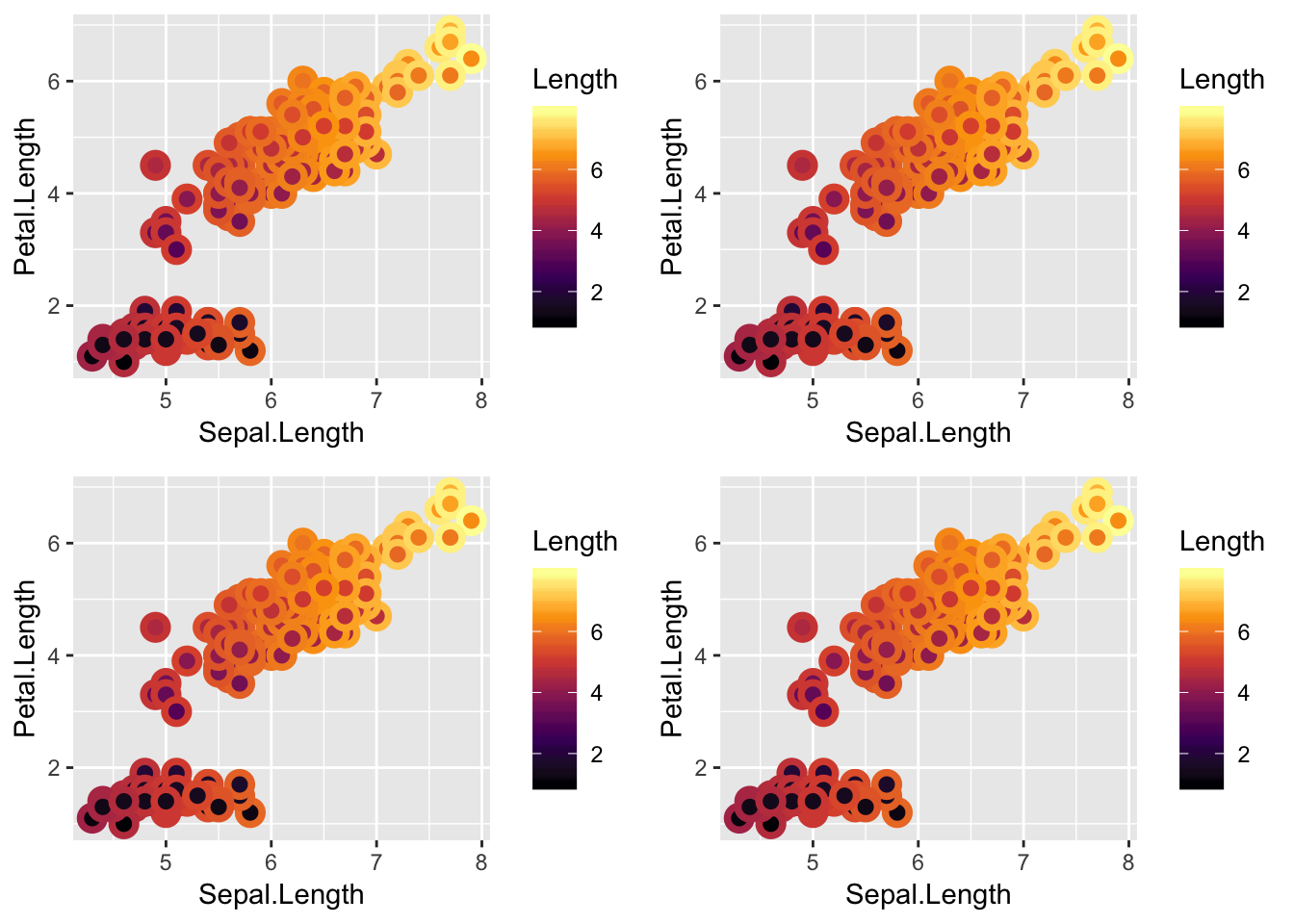Normalized statistics are now provided with `stat_bin2d()`, `stat_binhex()`, `stat_density_2d()`, and `stat_contour()` to plot 2d distributions normalized to a common height. This feature can be useful for faceted 2d plots, where the maximum density/count can vary greatly between panels. For example, when using `stat_density_2d()` with `contour = TRUE`, we can now use the calculated statistic `nlevel` to color by where each contour polygon lies relative to the highest one in that facet.

``````ggplot(diamonds, aes(x, depth)) +
stat_density_2d(
aes(fill = stat(nlevel)),
geom = "polygon",
n = 100,
bins = 10,
contour = TRUE
) +
facet_wrap(clarity~.) +
scale_fill_viridis_c(option = "A")
``````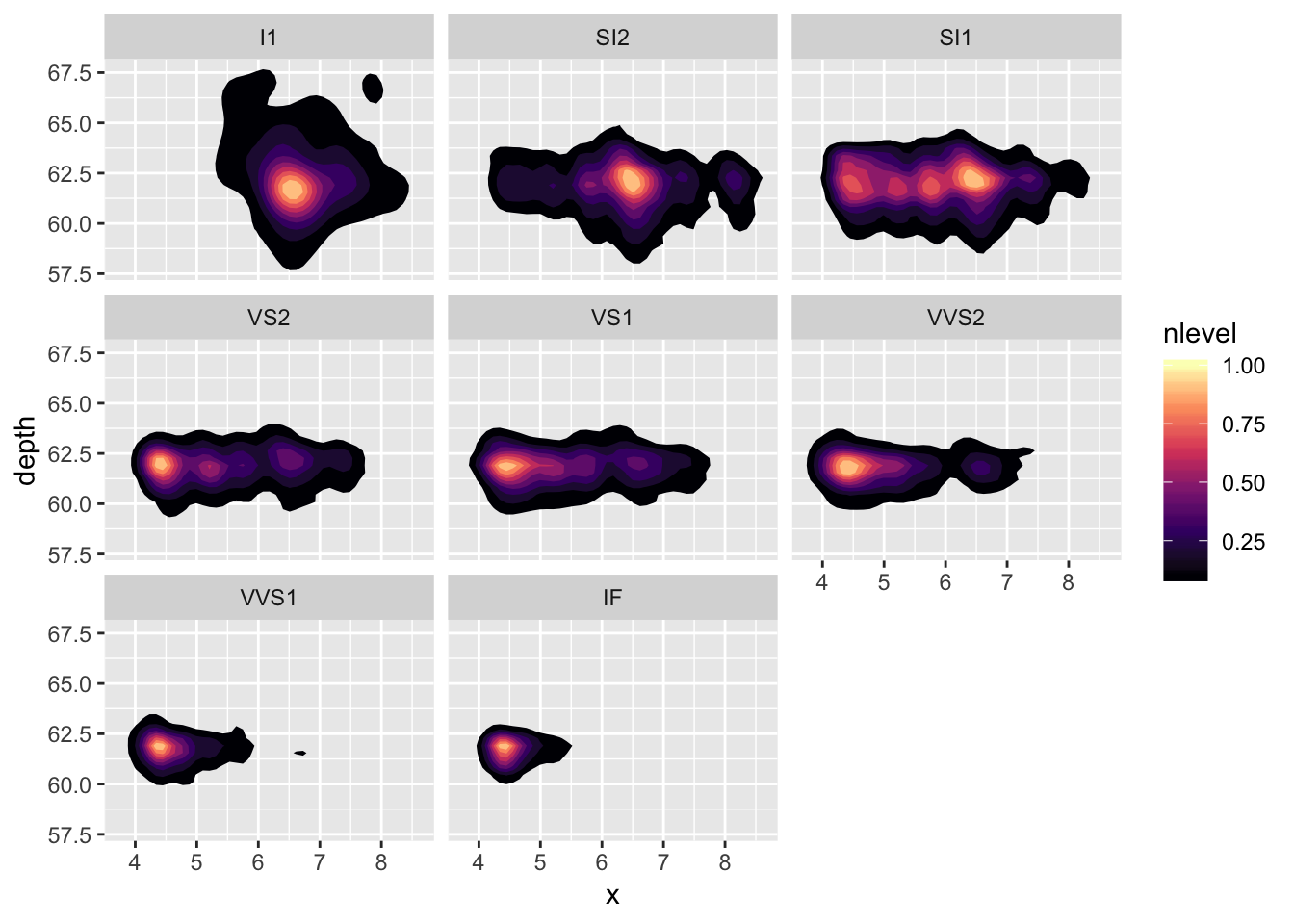Contents